Next: Empirical Evaluation Up: Efficiency Previous: Efficiency   Contents

Theoretical Considerations

In this section, we will see that although (single) round robin classification turns a single c-class learning problem into c(c-1)/2 two-class problems, the total training effort is only linear in the number of classes and smaller than the effort needed for an unordered binarization. The analysis is independent of the type of base learning algorithm used, although we will show that the advantage increases with the computational complexity of the algorithm. Some of the ideas have already been sketched in a short paragraph by Friedman (1996), but we go into considerably more detail and, in particular, focus on the comparison to conventional class binarization techniques.

In the following, we assume a base learner with a time complexity function f(n), i.e., the time needed for learning a classifier from a training set with n examples is f(n). Note that we interpret this as an exact function, and not as an asymptotically tight bound as in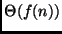because we are not interested in asymptotic behavior, but in the exact complexity at a given training set size n.8 We will consider functions of the form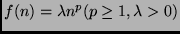and denote such a function with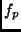. We use b|f to denote a class binarization with algorithm b, where an base learner with complexity f(n) is applied to each binary problem. Unless mentioned otherwise, all results refer to single round robin binarizations of problems with more than two classes (c > 2).

Definition 5.1 (class penalty)   Let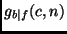be the total complexity for using a learner with complexity f(n) on a problem with c > 2 classes that has been class-binarized using binarization algorithm b. We then define the class penalty function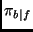as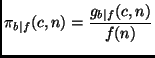Intuitively, the class penalty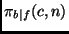measures the performance of an algorithm on a class binarized c-class problem relative to its performance on a single two-class problem the same size n. In the following, it will turn out that in some cases the class penalty function is independent of n or f. In such cases, we will abbreviate the notation as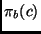.

Lemma 5.2 (class penalty for unordered class binarization)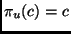Proof. There are c learning tasks, each using the entire training set of n examples. Hence the total complexity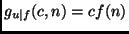, and.Lemma 5.3 (class penalty for single round robin, linear base algorithm)
For a base learner with a linear run-time complexity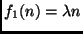:.

Proof. Each example of the original training set will occur exactly once in each of the c-1 binary tasks where its class is paired against one of the other c-1 classes. As there are n examples in the original training set, the total number of examples is (c-1)n.

As f is linear, the sum of the complexities on all individual training sets is equal to the complexity of the algorithm on the sum of all training set sizes, i.e.,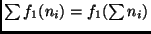. Hence,Therefore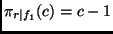.This analysis ignores a possible constant overhead of the algorithm, which potentially affects c(c-1)/2 function calls in the round robin case, while it only affects c function calls in the unordered case. However, some significant overhead costs, like reading in the training examples (and similar initialization steps) need, of course, only be performed once if the round robin procedure is performed in memory (which was not the case in the implementation which we used for the experimental results reported in the next section). If there is an overhead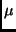to be considered (i.e.,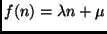), the total costs will be increased by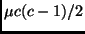. For very large values of c, these quadratic costs may outweigh the savings, but under reasonable assumptions (e.g.,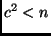) these additive costs should not matter, in particular--as we shall see in the following--not in the case of super-linear base algorithms.

Lemma 5.4 (class penalty for single round robin, super-linear base algorithm)
For p > 1: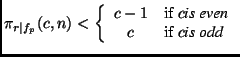Proof. Assume we have c classes, class i has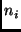examples,.

c is even:
In this case, we can arrange the learning tasks in the form of c-1 rounds. Each round consists of c/2 disjoint pairings, i.e., each class occurs exactly once in each round, and it has a different partner in each round. Such a tournament schedule is always possible.9 Without loss of generality, consider a round where classes 2i are paired against classes 2i-1. The complexity of this round is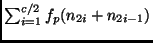. As for p > 1 and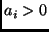, i = 1...N it holds that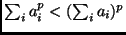, and because we assumed c > 2, we get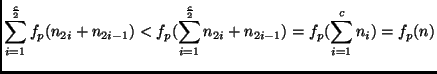Analogously, we can derive the same upper bound for each of the c-1 rounds. Thus the total complexity of the round robin binarization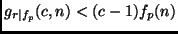and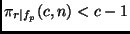.

c is odd:
we add a dummy class with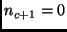examples, and perform a tournament as above. As this tournament has c rounds,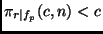.Note that the upper bounds in Lemma 5.4 are not tight (see also Theorems 5.6 and 5.7 below). In particular, the bound of c-1 should also hold for odd numbers but the proof for that case seems to be considerably more tricky.10 However, the current version suffices to prove the following theorem:

Theorem 5.5 (efficiency of round-robin and unordered binarization)
For algorithms with at least linear complexity (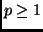):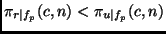, i.e., single round robin is more efficient than unordered binarization.

Proof. Follows immediately from Lemmas 5.25.3 and 5.4.As mentioned above, the bounds used for proving the lemmas are certainly not tight. This becomes obvious, if we look at problems with a uniform class distribution.

Theorem 5.6 (class penalty for class-balanced problems)
For a class-balanced problem,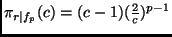Proof. In the (single) round robin case, we have c(c-1)/2 problems with 2n/c examples each. Hence the total complexity is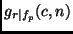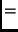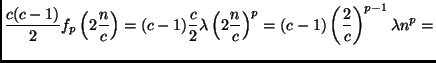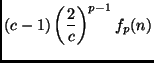Therefore.From this result, it is easy to see that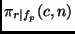decreases with increasing complexity order p of the base learner (assuming c > 2). Likewise, for p > 2,can be made arbitrarily small by increasing the number of classes c. While the latter property is hard to generalize for arbitrary class distributions--it is not the case that every problem with more than c classes has a smaller class penalty than an arbitrary c-class problem (and vice versa)--it is easy to prove the following theorem:

Theorem 5.7 (class penalty ratio for increasing order of base algorithm)
For c > 2, the class penalty ratio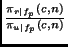is monotonically decreasing with p and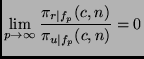Proof. The total effort for the single round robin is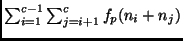. Hence the class penalty ratio is: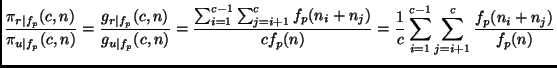Consequently,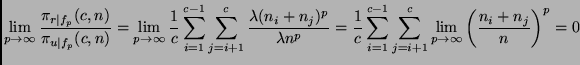The last equation holds because c > 2 and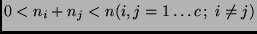, hence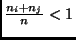. For the same reasons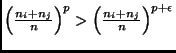for all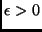andis monotonically decreasing with p.As the decrease with increasing order p is strictly monotonic, this theorem implies that the more expensive a learning algorithm is, the larger will be the efficiency gain for using round robin binarization instead of unordered binarization. In particular, it may be the case that for expensive algorithms, even a double round robin is faster than unordered binarization (in fact, it is easy to see that Theorem 5.7 also holds for double round robin binarization) or may be even faster than ordered binarization. Assume, e.g., an algorithm with a quadratic complexity on a class-balanced eight-class problem (i.e., p=2 and c = 8). According to Theorem 5.6 and Lemma 5.2: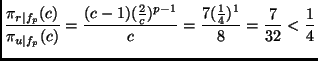Thus, under these circumstances, the single round robin is more than four times faster than the unordered approach. Considering that the double round robin is twice as slow as the single round robin, and assuming that the ordered approach is twice as fast as the unordered approach (see the following section for empirical values on that), the double round robin may in this case be faster than the ordered approach. This scenario will be empirically evaluated in the following section.Next: Empirical Evaluation Up: Efficiency Previous: Efficiency   Contents
Johannes Fürnkranz 2002-03-11